Next: Derivation and properties of Up: Darwin-Radau equation Previous: Introduction

# Calculation of gravitational potential

According to the analysis of Section 3.4, we can write(D.8)

where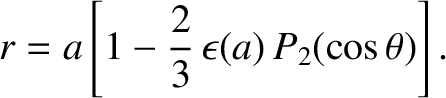(D.9)

Here, use has been made of Equation (D.5). Now, to first order in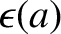, Equation (D.6) can be inverted to give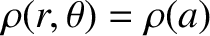(D.10)

Hence, to the same order, Equation (D.9) gives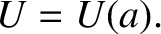(D.11)

Making use of Equation (3.42), we deduce that, to first order in,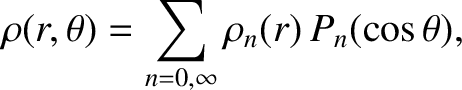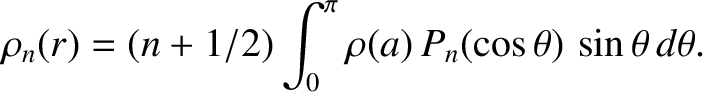(D.12) and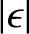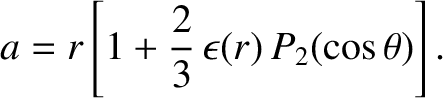(D.13)

with all of the other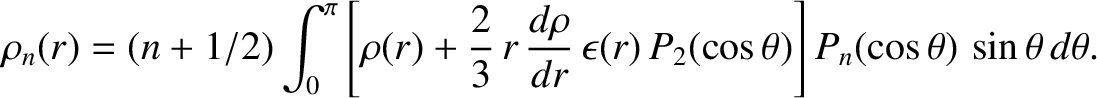zero.

The analysis of Section 3.4, combined with the previous two equations, also implies that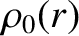(D.14)

where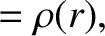(D.15)

and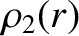(D.16)

Now, to first order in, we can write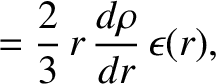(D.17)

where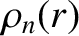(D.18)

Substitution of Equation (D.17) into Equations (D.15) and (D.16), followed by an expansion to first order in, yields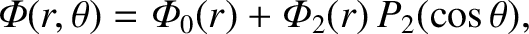(D.19)

where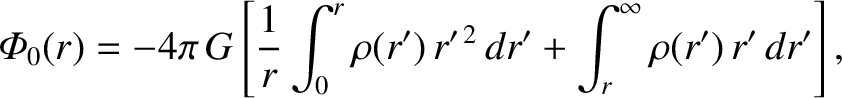(D.20)

and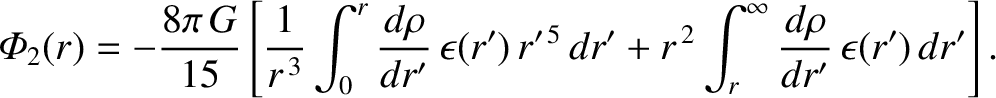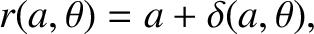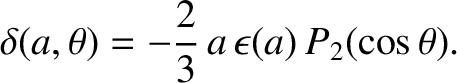(D.21)

Here, we have integrated the last two terms in curly brackets by parts.Next: Derivation and properties of Up: Darwin-Radau equation Previous: Introduction
Richard Fitzpatrick 2016-03-31